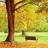# 标签：1+1=1 夜读1+1=1；1+2=1；3+4=1（绝了）文/一本叔主播/童童-01-1+1=1？有这样一个小故事：一所小学里，有个学生在作业本上写下了几个等式：1+1=1；1+2=1；3+4=1；5+7=1；6+1...
• 8月前
• 409 | 0